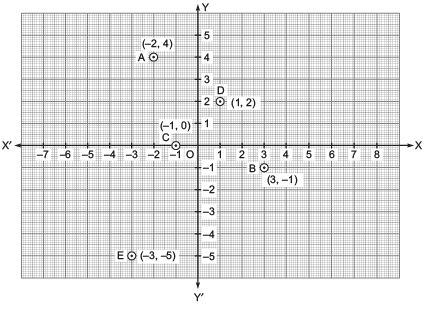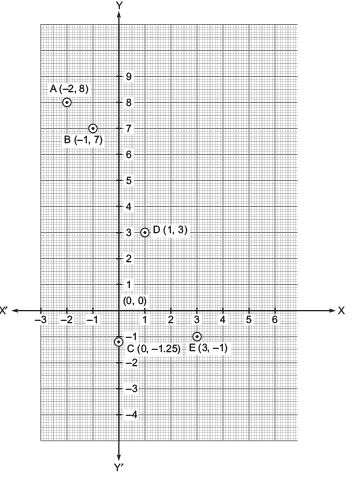Courses

# Ex 3.3 NCERT Solutions - Coordinate Geometry Class 9 Notes | EduRev

## Mathematics (Maths) Class 9

Created by: Full Circle

## Class 9 : Ex 3.3 NCERT Solutions - Coordinate Geometry Class 9 Notes | EduRev

The document Ex 3.3 NCERT Solutions - Coordinate Geometry Class 9 Notes | EduRev is a part of the Class 9 Course Mathematics (Maths) Class 9.
All you need of Class 9 at this link: Class 9

Question 1. In which quadrant or on which axis do each of the points (–2, 4) (3, –1), (–1, 0), (1, 2) and (–3, –5) lie? Verify your answer by locating them on the Cartesian plane.
Solution: In the point (–2, 4), the abscissa is negative and the ordinate is positive.
∵ (–, +) belongs to 2nd quadrant.
∴ (–2, 4) lies in the second quadrant.
The point (3, –1) is having +ve abscissa and –ve ordinate.
∴ (3, –1) lies in the 4th quadrant.
The point (–1, 0) is having (–ve) abscissa and ordinate on the x-axis.
∴ The point (–1, 0) lies on the negative x-axis.
The point (1, 2) is having the x-coordiante as well as y-coordinate +ve.
∴ Point (1, 2) lies in the 1st quadrant.
The point (–3, –5) is having the abscissa as well as ordinate negative.
∴ Point (–3, –5) lies in the 3rd quadrant.
These points are plotted in the Cartesian plane as shown in the following figure as A(–2, 4); B(3, –1); C(–1, 0); D(1, 2) and E(–3, –5).Question 2. Plot the points (x, y) given in the following table on the plane, choosing suitable units of distance on the axes.

 x -2 -1 0 1 3 y 8 7 -1.25 3 -1

Solution: The given points are (–2, 8), (–1, 7), (0, –1.5), (1, 3) and (3, –1). To plot these points:

(i) We draw X'OX and YOY' as axes.
(ii) We choose suitable units of distance on the axes.
(iii) To plot (–2, 8), we start from O, take (–2) units on x-axis and then (+8) units on y-axis.
We mark the point as A(–2, 8).
(iv) To plot (–1, 7), we start from 0; take (–1) units on x-axis and then (+7) units on the yaxis. We mark the point as B(–1, 7).
(v) To plot (0, –1.25), we move along 1.25 units below the x-axis on the y-axis and mark the point as C(0, –1.25).
(vi) To plot (1, 3), we take (+1) unit on the x-axis and then (+3) units on the y-axis. We mark the point as D(1, 3).
(vii) To plot (3, –1), we take (+3) units on the x-axis and then (–1) unit on the y-axis. We mark the point E(3, –1).,

,

,

,

,

,

,

,

,

,

,

,

,

,

,

,

,

,

,

,

,

;